Open in App
Not now

# Visualize data from CSV file in Python

• Difficulty Level : Hard
• Last Updated : 03 Mar, 2021

CSV stands for ‘Comma-Separated Values‘. It means the data(values) in a CSV file are separated by a delimiter i.e., comma. Data in a CSV file is stored in tabular format with an extension of .csv. Generally, CSV files are used with Google spreadsheets or Microsoft Excel sheets. A CSV file contains a number of records with the data spread across rows and columns. In this article, we are going to visualize data from a CSV file in Python.

To extract the data in CSV file, CSV module must be imported in our program as follows:

```import csv

with open('file.csv') as File:

Here, csv.reader( ) function is used to read the program after importing CSV library.

Example 1: Visualizing the column of different persons through bar plot.

The below CSV file contains different person name, gender, and age saved as ‘biostats.csv’: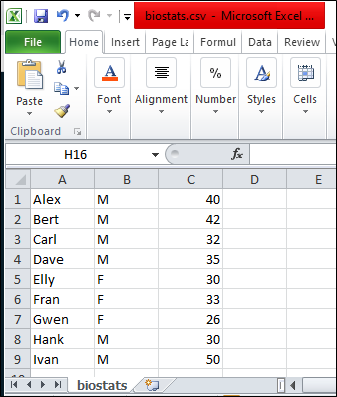The approach of the program:

1. Import required libraries, matplotlib library for visualizing, and CSV library for reading CSV data.
2. Open the file using open( ) function with ‘r’ mode (read-only) from CSV library and read the file using csv.reader( ) function.
3. Read each line in the file using for loop.
4. Append required columns into a list.
5. After reading the whole CSV file, plot the required data as X and Y axis.
6. In this example, we are plotting names as X-axis and ages as Y-axis.

Below is the implementation:

## Python3

 `import` `matplotlib.pyplot as plt``import` `csv`` ` `x ``=` `[]``y ``=` `[]`` ` `with ``open``(``'biostats.csv'``,``'r'``) as csvfile:``    ``plots ``=` `csv.reader(csvfile, delimiter ``=` `','``)``     ` `    ``for` `row ``in` `plots:``        ``x.append(row[``0``])``        ``y.append(``int``(row[``2``]))`` ` `plt.bar(x, y, color ``=` `'g'``, width ``=` `0.72``, label ``=` `"Age"``)``plt.xlabel(``'Names'``)``plt.ylabel(``'Ages'``)``plt.title(``'Ages of different persons'``)``plt.legend()``plt.show()`

Output :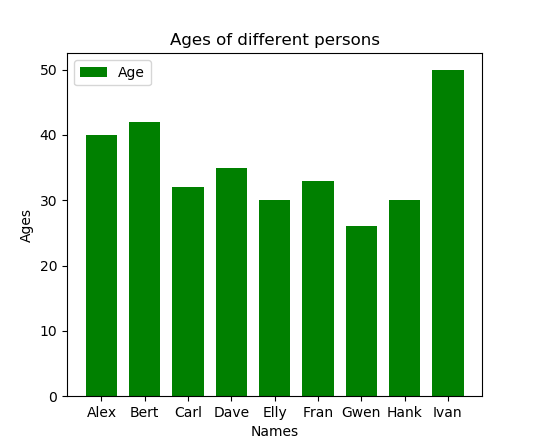Example 2: Visualizing Weather Report on different Dates” through-line plot.

Temperature(°C) on different dates is stored in a CSV file as ‘Weatherdata.csv’. These two rows ‘Dates’ and ‘Temperature(°C )’ are used as X and Y-axis for visualizing weather reports.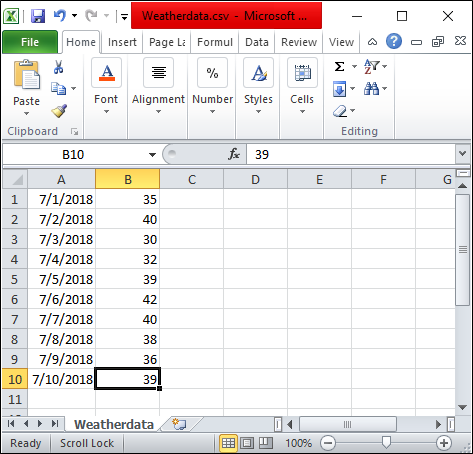Approach of the program:

1. Import required libraries, matplotlib library for visualizing, and csv library for reading CSV data.
2. Open the file using open( )  function with ‘r’ mode (read-only) from CSV library and read the file using csv.reader( ) function.
3. Read each line in the file using for loop.
4. Append required columns of the CSV file into a list.
5. After reading the whole CSV file, plot the required data as X and Y axis.
6. In this Example, we are plotting Dates as X-axis and Temperature(°C ) as Y-axis.

Below is the implementation:

## Python3

 `import` `matplotlib.pyplot as plt``import` `csv`` ` `x ``=` `[]``y ``=` `[]`` ` `with ``open``(``'Weatherdata.csv'``,``'r'``) as csvfile:``    ``lines ``=` `csv.reader(csvfile, delimiter``=``','``)``    ``for` `row ``in` `lines:``        ``x.append(row[``0``])``        ``y.append(``int``(row[``1``]))`` ` `plt.plot(x, y, color ``=` `'g'``, linestyle ``=` `'dashed'``,``         ``marker ``=` `'o'``,label ``=` `"Weather Data"``)`` ` `plt.xticks(rotation ``=` `25``)``plt.xlabel(``'Dates'``)``plt.ylabel(``'Temperature(°C)'``)``plt.title(``'Weather Report'``, fontsize ``=` `20``)``plt.grid()``plt.legend()``plt.show()`

Output :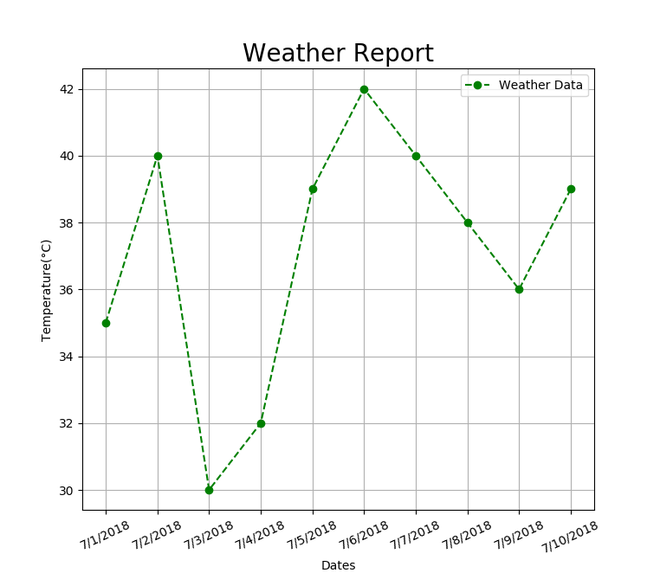Example 3: Visualizing patients blood pressure report of a hospital through Scatter plot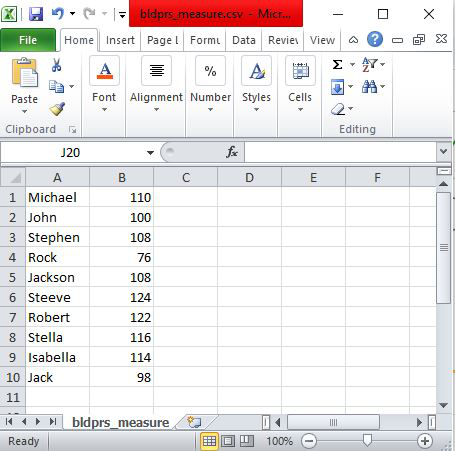Approach of the program “Visualizing patients blood pressure report” through Scatter plot :

1. Import required libraries, matplotlib library for visualization and importing csv library for reading CSV data.
2. Open the file using open( ) function with ‘r’ mode (read-only) from CSV library and read the file using csv.reader( ) function.
3. Read each line in the file using for loop.
4. Append required columns of the CSV file into a list.
5. After reading the whole CSV file, plot the required data as X and Y axis.
6. In this example, we are plotting the Names of patients as X-axis and Blood pressure values as Y-axis.

Below is the implementation:

## Python3

 `import` `matplotlib.pyplot as plt``import` `csv`` ` `Names ``=` `[]``Values ``=` `[]`` ` `with ``open``(``'bldprs_measure.csv'``,``'r'``) as csvfile:``    ``lines ``=` `csv.reader(csvfile, delimiter``=``','``)``    ``for` `row ``in` `lines:``        ``Names.append(row[``0``])``        ``Values.append(``int``(row[``1``]))`` ` `plt.scatter(Names, Values, color ``=` `'g'``,s ``=` `100``)``plt.xticks(rotation ``=` `25``)``plt.xlabel(``'Names'``)``plt.ylabel(``'Values'``)``plt.title(``'Patients Blood Pressure Report'``, fontsize ``=` `20``)`` ` `plt.show()`

Output :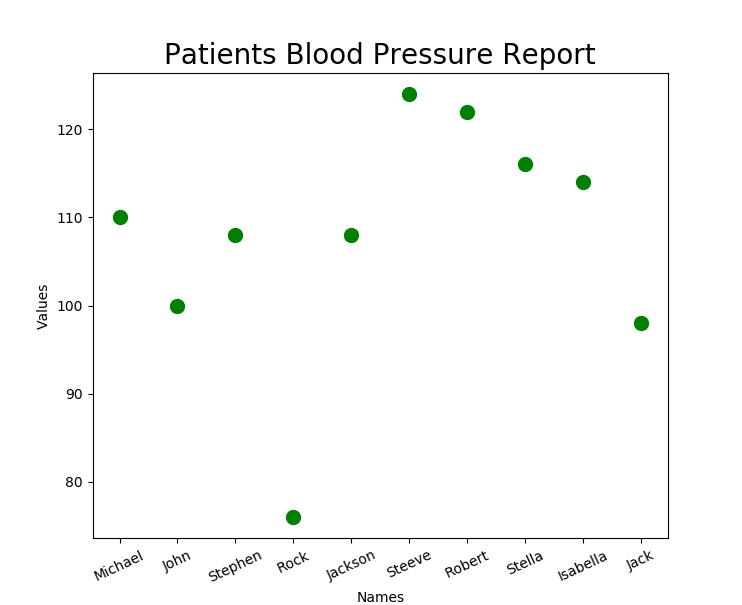Example 4: Visualizing Student marks in different subjects using a pie plotApproach of the program:

1. Import required libraries, matplotlib library for visualization and importing csv library for reading CSV data.
2. Open the file using open( )  function with ‘r’ mode (read-only) from CSV library and read the file using csv.reader( ) function.
3. Read each line in the file using for loop.
4. Append required columns of the CSV file into lists.
5. After reading the whole CSV data, plot the required data as pie plot using plt.pie( ) function.

Below is the implementation:

## Python3

 `import` `matplotlib.pyplot as plt``import` `csv`` ` `Subjects ``=` `[]``Scores ``=` `[]`` ` `with ``open``(``'SubjectMarks.csv'``, ``'r'``) as csvfile:``    ``lines ``=` `csv.reader(csvfile, delimiter ``=` `','``)``    ``for` `row ``in` `lines:``        ``Subjects.append(row[``0``])``        ``Scores.append(``int``(row[``1``]))`` ` `plt.pie(Scores,labels ``=` `Subjects,autopct ``=` `'%.2f%%'``)``plt.title(``'Marks of a Student'``, fontsize ``=` `20``)``plt.show()`

Output :My Personal Notes arrow_drop_up